##### Geometry: 1,001 Practice Problems For Dummies (+ Free Online Practice)You can identify triangles by their angle measurements. Using the fact that all angles in a triangle add up to 180 degrees, you apply some algebra to determine whether a triangle is equiangular, isosceles, scalene, right, acute, or obtuse.

## Practice questions

Triangle WXY is drawn with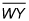extended to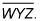Use the diagram and the given information to classify each triangle as acute, obtuse, equiangular, or right.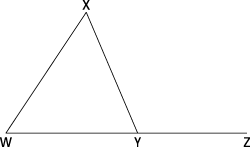1.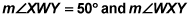is 20 more than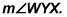2.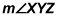is 30 more than twice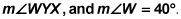1. Acute

Write down the given angle measures: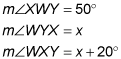The sum of the three angles of a triangle is 180 degrees: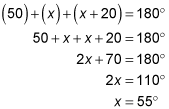Find the measure of each angle by plugging in 55 degrees for x: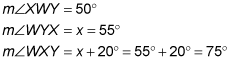Because the largest angle of this triangle is less than 90 degrees, this triangle is acute.

2. Right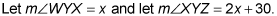These two angles form a linear pair, so they add up to 180 degrees. Use these relationships to solve for x and find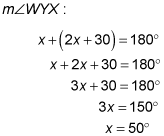Therefore,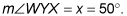With this info and the given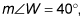find the measure of the remaining angle in the triangle: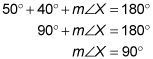If one angle in the triangle is 90 degrees, then the triangle is a right triangle.